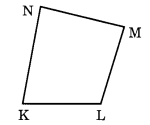NCERT Solutions for Class 6 Maths Chapter 4 Basic Geometrical Ideas Ex 4.5

# NCERT Solutions for Class 6 Maths Chapter 4 Basic Geometrical Ideas Ex 4.5

## NCERT Solutions for Class 6 Maths Chapter 4 Basic Geometrical Ideas Ex 4.5

NCERT Solutions for Class 6 Maths Chapter 4 Basic Geometrical Ideas Ex 4.5 are the part of NCERT Solutions for Class 6 Maths. Here you can find the NCERT Solutions for Class 6 Maths Chapter 4 Basic Geometrical Ideas Ex 4.5.

### Ex 4.5 Class 6 Maths Question 1.

Draw a rough sketch of a quadrilateral PQRS. Draw its diagonals. Name them. Is the meeting point of the diagonals in the interior or exterior of the quadrilateral?

Solution:

(i) We have drawn a quadrilateral PQRS.
(ii) We have drawn two diagonals PR and QS.
(iii) We can see that O is the meeting point of the diagonals PR and QS which is in the interior of the quadrilateral.

### Ex 4.5 Class 6 Maths Question 2.

Draw a rough sketch of a quadrilateral KLMN. State:
(a) two pairs of opposite sides,
(b) two pairs of opposite angles,
(c) two pairs of adjacent sides,
(d) two pairs of adjacent angles.

Solution:
We have drawn a quadrilateral KLMN as given below.(a) (KLNM) and (KNLM) are the two pairs of opposite sides.
(b) (
K, M) and (L, N) are the two pairs of opposite angles.
(c) (KL, KN) and (NM, ML) are the two pairs of adjacent sides.
(d) (
K, L) and (N, M) are the two pairs of adjacent angles.

You can also like these:

NCERT Solutions for Maths Class 7

NCERT Solutions for Maths Class 8

NCERT Solutions for Maths Class 9

NCERT Solutions for Maths Class 10

NCERT Solutions for Maths Class 11

NCERT Solutions for Maths Class 12